﻿ 基于数字全息显微技术的生物细胞动态定量测量 Dynamic Quantitative Measurement of Cells Based on Digital Holographic Microscopy

Optoelectronics
Vol. 09  No. 02 ( 2019 ), Article ID: 30575 , 6 pages
10.12677/OE.2019.92012

Dynamic Quantitative Measurement of Cells Based on Digital Holographic Microscopy

Kangyan Su, Yanan Zeng*, Yuan Liu, Haiyun Wu, Xiayu Chen

College of Engineering and Technology, Tianjin Agricultural University, Tianjin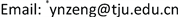Received: May 13th, 2019; accepted: May 24th, 2019; published: May 31st, 2019ABSTRACT

The dynamic measurement of biological cells has been based on the qualitative observations for a long time. As a transparent sample, a cell’s optical path length is linearly related to cell morphology and cytoplasm concentration. As a result, the living state of a cell can be reflected by the optical path length quantitatively. Optical path length can be obtained by digital holographic microscopy without damaging the sample. In this paper, we used the transmission digital holographic microscopy system to observe the HeLa cells and Escherichia coli dynamically to obtain the optical path lengths. By analyzing the relationship between optical path lengths and living state of biological cells, a new quantitative measurement method is proposed to explore the influences caused by the environment.

Keywords:Digital Holographic Microscopy, Optical Path Length, Dynamic Measurement1. 引言

2. 测量系统与测量原理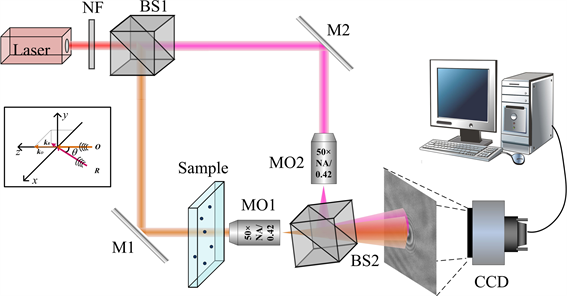Laser：激光光源；NF：中灰镜；BS1，BS2：分光棱镜；M1，M2：反射镜；MO1，MO2：显微物镜。

Figure 1. Schematic of transmission digital holographic microscope

$\begin{array}{l}I\left({x}_{h},{y}_{h}\right)={|U\left({x}_{h},{y}_{h}\right)+R\left({x}_{h},{y}_{h}\right)|}^{2}\\ =U{\left({x}_{h},{y}_{h}\right)}^{2}+R{\left({x}_{h},{y}_{h}\right)}^{2}+{U}^{*}\left({x}_{h},{y}_{h}\right)R\left({x}_{h},{y}_{h}\right)+U\left({x}_{h},{y}_{h}\right){R}^{*}\left({x}_{h},{y}_{h}\right)\end{array}$ (1)

$\left({x}_{h},{y}_{h}\right)$ 是全息面的坐标。 $U\left({x}_{h},{y}_{h}\right)$$R\left({x}_{h},{y}_{h}\right)$ 分别是传播到全息面的物光波和参考光波的复振幅。*代表复共轭。因此重构物光波的复振幅过程为

$U\left({x}_{r},{y}_{r}\right)=A\cdot {\mathcal{F}}^{-1}\left\{D\left({f}_{{x}_{h}},{f}_{{y}_{h}},{x}_{h},{y}_{h}\right)W\left({f}_{{x}_{h}},{f}_{{y}_{h}}\right)\mathcal{F}\left(I\left({x}_{h},{y}_{h}\right)\right)\mathrm{exp}\left[-i\text{π}\lambda d\left({\nu }_{{x}_{h}}^{2}+{\nu }_{{y}_{h}}^{2}\right)\right]\right\}$ (2)

$\varphi \left({x}_{r},{y}_{r}\right)=\mathrm{arctan}\left\{\frac{\mathrm{Im}\left(U\left({x}_{r},{y}_{r}\right)\right)}{\mathrm{Re}\left(U\left({x}_{r},{y}_{r}\right)\right)}\right\}$ (3)

${L}_{OP}=\frac{\varphi \lambda }{2\text{π}}$ (4)

3. 实验系统与测量结果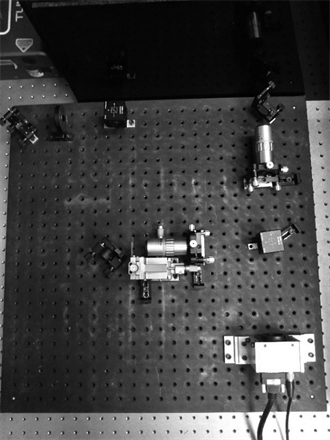Figure 2. Setup of transmission digital holographic microscope(a)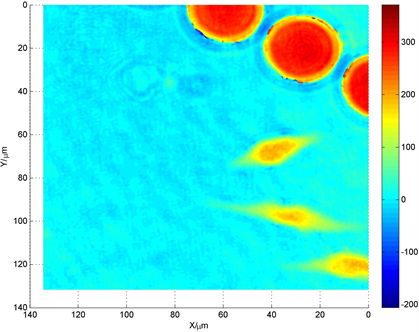(b)

Figure 3. (a) Digital hologram; (b) The reconstructed optical path length of Hela cells in the micro-fluid chip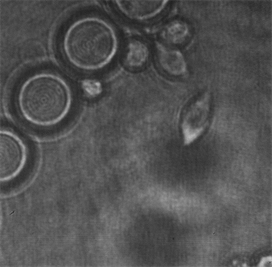(a)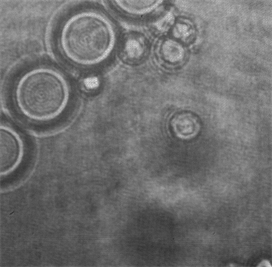(b)

Figure 4. The image-plane hologram of (a) Living and (b) Dead Hela cells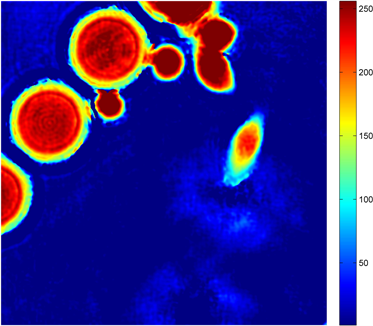(a)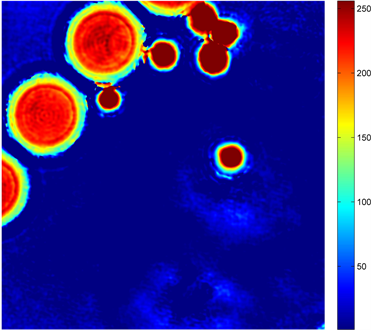(b)

Figure 5. Reconstructed optical path length of (a) Living and (b) Dead Hela cells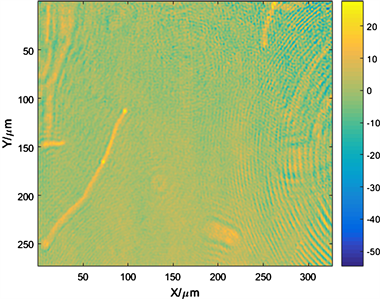(a)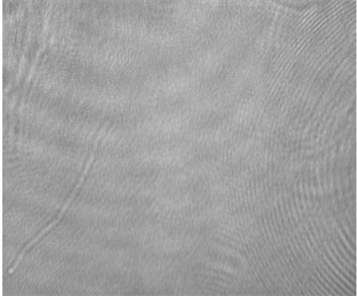(b)

Figure 6. (a) Reconstructed optical path length and (b) phase of Escherichia coli.

4. 结论

Dynamic Quantitative Measurement of Cells Based on Digital Holographic Microscopy[J]. 光电子, 2019, 09(02): 77-82. https://doi.org/10.12677/OE.2019.92012

1. 1. Mann, C., Yu, L., Lo, C.-M. and Kim, M. (2005) High-Resolution Quantitative Phase-Contrast Microscopy by Digital Holography. Optics Express, 13, 8693-8698. https://doi.org/10.1364/OPEX.13.008693

2. 2. Khmaladze, A., Kim, M. and Lo, C.-M. (2008) Phase Imaging of Cells by Simultaneous Dual-Wavelength Reflection Digital Holography. Optics Express, 16, 10900-10911. https://doi.org/10.1364/OE.16.010900

3. 3. Mann, C.J., Yum, L. and Kim, K. (2006) Movies of Cellular and Sub-Cellular Motion by Digital Holographic Microscopy. BioMedical Engineering OnLine, 5, 21.

4. 4. Charrière, F., Pavillon, N., Colomb, T., et al. (2006) Living Specimen Tomography by Digital Holographic Microscopy: Morphometry of Testate Amoeba. Optics Express, 14, 7005-7013. https://doi.org/10.1364/OE.14.007005

5. 5. Debailleul, M., Simon, B., Georges, V., et al. (2008) Holographic Microscopy and Diffractive Microtomography of Transparent Samples. Measurement Science and Technology, 19, 074009. https://doi.org/10.1088/0957-0233/19/7/074009

6. 6. Rappaz, B., Barbul, A., Hoffmann, A., et al. (2009) Spatial Analysis of Erythrocyte Membrane Fluctuations by Digital Holographic Microscopy. Blood Cells, Molecules, and Diseases, 42, 228-232. https://doi.org/10.1016/j.bcmd.2009.01.018

7. 7. Kemper, B., Carl, D., Schnekenburger, J., et al. (2006) Investigation of Living Pancreas Tumor Cells by Digital Holographic Microscopy. Journal of Biomedical Optics, 11, 034005. https://doi.org/10.1117/1.2204609

8. 8. Yu, L.F., Mohanty, S., Zhang, J., et al. (2009) Digital Holographic Microscopy for Quantitative Cell Dynamic Evaluation during Laser Microsurgery. Optics Express, 17, 12031-12038. https://doi.org/10.1364/OE.17.012031

9. 9. Christophe, M., Natacha, C., Gwennou, C., et al. (2008) Fast Measurements of Concentration Profiles inside Deformable objects in Microflows with Reduced Spatial Coherence Digital Holography. Applied Optics, 47, 5305-5314. https://doi.org/10.1364/AO.47.005305

NOTES

*通讯作者。